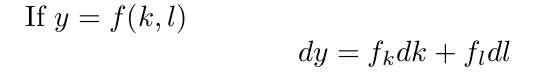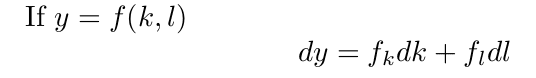# What exactly is a 'total derivative'?

## Homework Statement

What exactly is a total derivative? What is the definition of this concept?

## Homework Equations

An example of total derivatives:## The Attempt at a Solution

I've tried searching for it, but found no helpful information.

SammyS
Staff Emeritus
Homework Helper
Gold Member

## Homework Statement

What exactly is a total derivative? What is the definition of this concept?

## Homework Equations

An example of total derivatives:## The Attempt at a Solution

I've tried searching for it, but found no helpful information.
What you have is a differential, not a derivative.

That's the differential in a general form. For a specific point you evaluate the partial derivatives on the right side at the point and then you have a linear function of dk and dl.

## Homework Statement

What exactly is a total derivative? What is the definition of this concept?

## Homework Equations

An example of total derivatives:## The Attempt at a Solution

I've tried searching for it, but found no helpful information.

You may contrast your "total derivative", with partial derivative. The total is sort of an absolute change, like if you have a (k,l)(t) path, and head in the (dk,dl) direction. You can contrast this with the partial derivatives, fk and fl, which would contribute to parts of the total derivative. Or, you might have a path k(l), so you could find the total derivative along that path. Another definition of total derivative, would be the vector (fk,fl), otherwise known as the gradient. The tricky part is, the various realizations of the term total derivative can be a little confusing, I don't think I happened to notice all this until I graduated and had a chance to look back at all the contexts I had seen it.

A total derivative is taking the derivatives of a function wrt all variables. In the case of steady fluid dynamics, this would be the partials with respect to X Y and Z. As mentioned earlier, this is also known as taking the gradient of a function.

You may contrast your "total derivative", with partial derivative. The total is sort of an absolute change, like if you have a (k,l)(t) path, and head in the (dk,dl) direction. You can contrast this with the partial derivatives, fk and fl, which would contribute to parts of the total derivative. Or, you might have a path k(l), so you could find the total derivative along that path. Another definition of total derivative, would be the vector (fk,fl), otherwise known as the gradient. The tricky part is, the various realizations of the term total derivative can be a little confusing, I don't think I happened to notice all this until I graduated and had a chance to look back at all the contexts I had seen it.

I was hoping to pull together the various definitions of total derivative from
http://en.wikipedia.org/wiki/Total_derivative
It includes Jacobian, gradient (special case of Jacobian), and, somehow consistent with that, a gradient dotted with a path vector, which could be parametrized by one of the original independent variables. I suppose the latter is like the former in that it is the total change as we move along in t, or in a way, in x, so we contrast df/dx with ∂f/∂x, another strangeness.